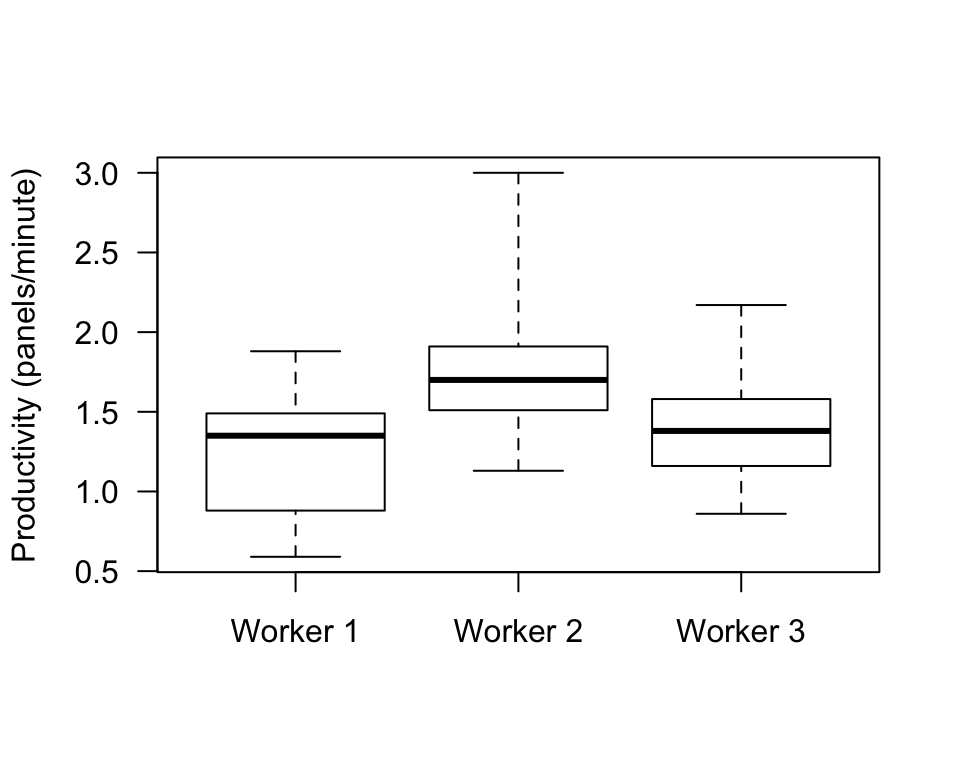## D.13 Answers: Numerical summaries for quantitative data

Answers to exercises in Sect. 13.10.

Answer to Exercise 13.1: Probably the median as slightly skewed right, with some outliers. Both the mean and median can be quoted…
Answer to Exercise 13.2: 1. Sample mean: 0.467. 2. Sample median: 3.35. 3. Range: 29.6 (from -19.8 to 9.8). 4. Sample standard deviation: 10.40263. (SOI has no units of measurement.)
Answer to Exercise 13.3: A: II (median; IQR). B: I (mean; standard deviation). C: III (median; IQR).
Answer to Exercise 13.4: See Fig D.5. Worker 2 is faster in general (more panels installed per minute), including one fast outlier. Workers 1 and 3 have similar medians, but Worker 3 is more consistent (smaller IQR).FIGURE D.5: The boxplot for the productivity data